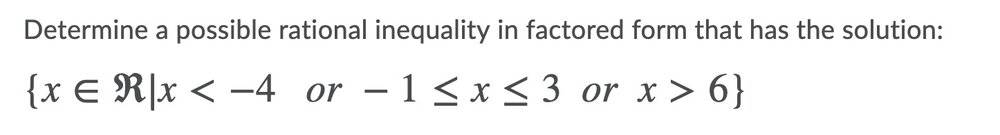# Rational inequalities homework help please

• MartynaJ
In summary, the conversation discusses solving polynomial inequalities by working backwards and constructing the polynomial given the solution. It is suggested to start by describing the steps to solve the inequality and then applying them in reverse. For rational expressions, it is important to note that the sign of f(x) and 1/f(x) are the same, so the denominator can be omitted in most cases.f

#### MartynaJ

Homework Statement
Determine a possible rational inequality in factored form with the solution ##x<-4## or ##-1\leq x\leq 3## or ##x>6##
Relevant EquationsMy attempt so far:
I put all the terms to become smaller than zero:
so ##x<-4## becomes ##x-4<0##
##-1\leq x\leq 3## becomes ##-1-x\leq 0## and ##x-3 \leq 0##
##x>6## becomes ##x-6>0## which is the same as ##-x+6<0## (i think)...

I am now stuck on making it a rational inequality... anyone know how?

Thanks

If you have a polynomial inequality, like ##x^2-4 < 0##, do you know how to solve it? I think the idea here is to do this process in reverse, and construct the polynomial given the solution.

Also x < -4 becomes x+4 < 0.

•Delta2
If you have a polynomial inequality, like ##x^2-4 < 0##, do you know how to solve it? I think the idea here is to do this process in reverse, and construct the polynomial given the solution.

Also x < -4 becomes x+4 < 0.
Ya x < -4 becomes x+4 < 0 (that was a typo)... and yes the idea in this question is to do it in reverse (i.e. try to find the polynomial)... I am just unsure of how to do that exactly.

Why don't you start by describing the steps you would do to solve it the other way, then we can figure out how to work backwards.

Why don't you start by describing the steps you would do to solve it the other way, then we can figure out how to work backwards.
For a polynomial inequality, I would just need to multiply them together. I'm not sure how to find make a rational expression. Like how do I know what to put in the numerator and what to put in thre denominator?

Multiply what together? Can you solve the expression ##x^2-4 < 0##? I promise you if you just write out how to solve it, it will be easy to show how to do the steps in reverse.

As far as the rational expression, note the sign of f(x) and 1/f(x) are the same, so you can mostly just use a ppolynomial and not worry about putting anything in the denominator.

For a polynomial inequality, I would just need to multiply them together. I'm not sure how to find make a rational expression. Like how do I know what to put in the numerator and what to put in thre denominator?
If a factor such as ##x-3## is in the denominator, then can ##x## be equal to three? Why not?